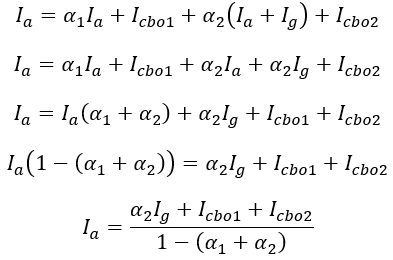# Two Transistor Model of SCR or Thyristor – Circuit Diagram & Analysis

Two transistor model is used to explain the principle of operation of the thyristor in a very simple way. Since a thyristor is a four-layered PNPN device, it can be considered as a combination of two transistors, one transistor as PNP and the other transistor as NPN. A two-transistor model is obtained by separating the two middle layers of the thyristor into two parts as shown in the below figure.

In this model, base current Ib1 of transistor Q1 is equal to the collector current Ic2 of transistor Q2 and vice-versa. The equivalent circuit of the two transistor analogy is shown below.

#### From transistor analysis, we have,Ie1 = Ib1 + Ic1Ib1 = Ie1 – Ic1 …(7)Substituting equation 2 in equation 6, we get,If the leakage currents Icbo1 and Icbo2 of transistors Q1 and Q2 are negligibly small, then,

From the above equation, it can be analyzed that, if α1 + α1 = 1, then anode current Ia becomes infinity and hence thyristor enters into conduction state from non-conduction state. In other words, if Ig reaches a value such that α1 + α2 = 1, then SCR is turned ON and this action is called the Regenerative action.